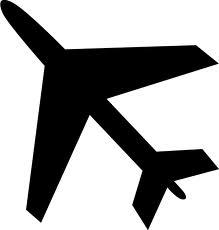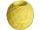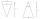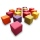# Shape

Plane shape has a maximum area 677 mm2. Calculate its perimeter if perimeter is the smallest possible.

o =  92.2 mm

### Step-by-step explanation:Did you find an error or inaccuracy? Feel free to write us. Thank you!#### You need to know the following knowledge to solve this word math problem:

We encourage you to watch this tutorial video on this math problem:

## Related math problems and questions:

• Circle - simpleThe circumference of a circle is 198 mm. How long in mm is its diameter?
• CircleHow big is an area of a circle if its circumference is 51.2 cm?
• Square s3Calculate the diagonal of the square, where its area is 0.49 cm square. And also calculate its circumference.Find the radius of the circle with area S = 200 cm².
• The schoolyardThe schoolyard had the shape of a square with an 11m side. The yard has been enlarged by 75 m2 and has a square shape again. How many meters was each side of the yard enlarged?
• Circle from stringMartin has a long 628 mm string. He makes a circle from it. Calculate the radius of the circle.
• The cubeThe cube has a surface area of 486 m ^ 2. Calculate its volume.
• Four circles1) Calculate the circle radius if its area is 400 cm square 2) Calculate the radius of the circle whose circumference is 400 cm. 3) Calculate circle circumference if its area is 400 cm square 4) Calculate the circle's area if perimeter 400 cm.
• Perimeter to areaCalculate the area of a circle with a perimeter of 15 meters.
• TerezaThe cube has an area of base 256 mm2. Calculate the edge length, volume, and area of its surface.
• Area to perimeterCalculate circle circumference if its area is 254.34cm2
• Largest possible coneIt is necessary to make the largest possible cone from an iron rod in the shape of a prism with dimensions of 5.6 cm, 4.8 cm, 7.2 cm. a) Calculate its volume. b) Calculate the waste.
• Quarter of a circleCalculate the circumference of a quarter circle if its content is S = 314 cm2.
• Two wallsCalculate the surface area of a cube in m2 if you know that the area of its two walls is 72 dm2.
• Tripled squareIf you tripled the length of the sides of the square ABCD you increases its content by 200 cm2. How long is the side of the square ABCD?Calculate the radius of the circle whose length is 107 cm larger than its diameterThe circle has a radius 6 cm. Calculate: